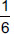# User Forum

Subject :IMO    Class : Class 9

ABCD is parallelogram, G is the point on AB such that AG = 2GB, E is point on DC such that CE = 2DE and F is the point on BC such that BF = 2FC. Then, match the following:

 Column-I Column-II P. ar (ADEG) (i)ar (ABCD) Q. ar (∆EGB) (ii) ar (∆EDG) R. ar (∆EFC) (iii) ar (GBCE) S. ar (∆EGB) (iv)ar (∆EBF)

 P Q R S A (i) (ii) (iii) (iv) B (iii) (i) (iv) (ii) C (iii) (ii) (iv) (i) D (ii) (i) (iii) (iv)

Why cant it be C